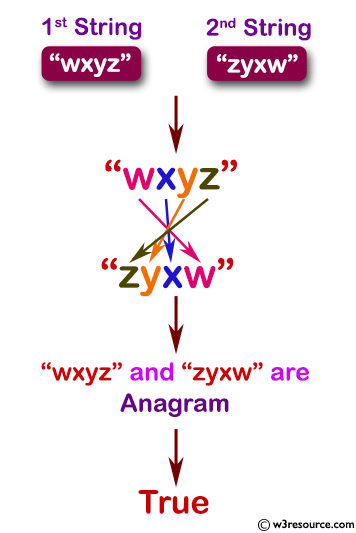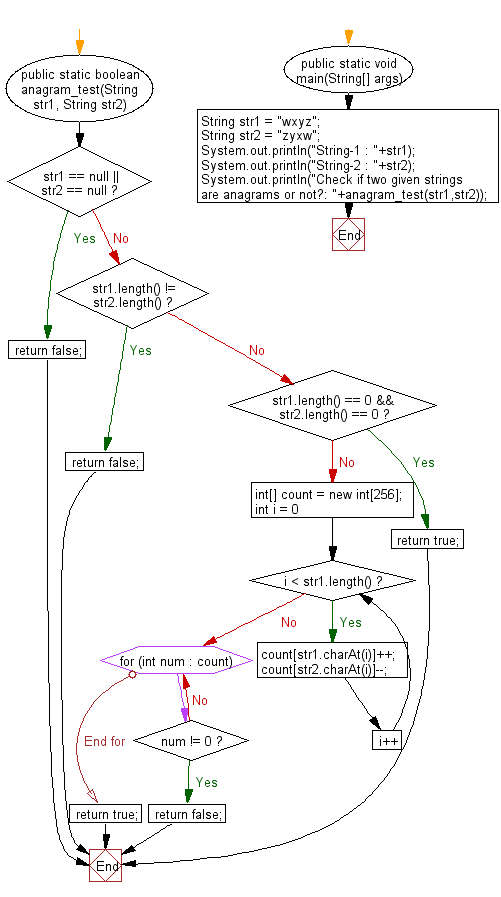﻿ Java exercises: Check if two given strings are anagrams or not - w3resource# Java Exercises: Check if two given strings are anagrams or not

## Java Basic: Exercise-142 with Solution

Write a Java program to check if two given strings are anagrams or not.

According to wikipedia "An anagram is a word or phrase formed by rearranging the letters of a different word or phrase, typically using all the original letters exactly once. For example, the word anagram can be rearranged into nag a ram, or the word binary into brainy."

Pictorial Presentation:Sample Solution:

Java Code:

``````public  class Solution {
/**
* @param s: The first string
* @param b: The second string
* @return true or false
*/
public static boolean anagram_test(String str1, String str2) {
if (str1 == null || str2 == null) {
return false;
} else if (str1.length() != str2.length()) {
return false;
} else if (str1.length() == 0 && str2.length() == 0) {
return true;
}
int[] count = new int;
for (int i = 0; i < str1.length(); i++) {
count[str1.charAt(i)]++;
count[str2.charAt(i)]--;
}
for (int num : count) {
if (num != 0) {
return false;
}
}
return true;
}
public static void main(String[] args) {
String str1 = "wxyz";
String str2 = "zyxw";
System.out.println("String-1 : "+str1);
System.out.println("String-2 : "+str2);
System.out.println("Check if two given strings are anagrams or not?: "+anagram_test(str1,str2));
}
}
```
```

Sample Output:

```String-1 : wxyz
String-2 : zyxw
Check if two given strings are anagrams or not?: true
```

Flowchart:Java Code Editor:

Company:

What is the difficulty level of this exercise?

Test your Programming skills with w3resource's quiz.

﻿

## Java: Tips of the Day

getEnumMap

Converts to enum to Map where key is the name and value is Enum itself.

```public static <E extends Enum<E>> Map<String, E> getEnumMap(final Class<E> enumClass) {
return Arrays.stream(enumClass.getEnumConstants())
.collect(Collectors.toMap(Enum::name, Function.identity()));
}
```

Ref: https://bit.ly/3xXcFZt